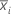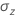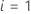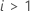# EWMA chart

Select the method or formula of your choice.

## Plotted points

By changing the weight used and the number of σ's for the control limits, you can construct a chart with specific properties. Combinations of these two parameters are often chosen by using an ARL (average run length) table. See Lucas et al.1 for the table of average run lengths.

### Notation

TermDescription
rweightmean of subgroup i

## Center line

The center line represents the process average, μ. If you do not specify an historical value for μ, then Minitab uses the mean of all observations,, to estimate μ.

## Control limits

If you do not specify a historical value for the process standard deviation, σ, then Minitab estimates σ from your data using the specified method.

### Standard deviation of the plotted pointsFor the first subgroup (),is calculated as follows:For subsequent subgroups (),is calculated as follows:

When subgroup sizes are constant, the equation simplifies to the following:

### Notation

TermDescription
nisize of subgroup i
rweight of EWMA
μprocess mean
kparameter for Test 1. The default is 3.
1 J.M. Lucas and M.S. Saccucci (1990). "Exponentially Weighted Moving Average Control Schemes: Properties and Enhancements," Technometrics, 32, 1−12.
By using this site you agree to the use of cookies for analytics and personalized content.  Read our policy